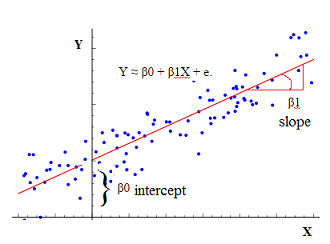# Statistics - Cross Product (of X and Y) (CP|SP)

The cross product is a calculation used in order to define the correlation coefficient between two variables.

SP is the sum of all cross products between two variables.

## Computation

### CP

For one observation (one row), multiply the deviation score on the variable X by the deviation score on the variable Y:

$$\text{Cross Product}=(\href{raw_score}{X}_i-\href{mean}{\bar{X}}).(\href{raw_score}{Y}_i-\href{mean}{\bar{Y}})$$

### SP

SP is the sum of cross product between two variables (X and Y) for all observations. $$\begin{array}{rrl} \text{Sum of Cross Product (SP)} & = & \sum_{i=1}^{\href{sample_size}{N}}{\href{cross_product}{\text{Cross Product of X & Y}}} \\ & = & \sum_{i=1}^{\href{sample_size}{N}}{(\href{raw_score}{X}_i-\href{mean}{\bar{X}}).(\href{raw_score}{Y}_i-\href{mean}{\bar{Y}})} \\ \end{array}$$

Recommended PagesStatistics - (Univariate|Simple|Basic) Linear Regression

A Simple Linear regression is a linear regression with only one predictor variable (X). Correlation demonstrates the relationship between two variables whereas a simple regression provides an equation...Statistics - Correlation (Coefficient analysis)

Correlation is a statistical analysis used to measure and describe the relationship betweentwo variables. The Correlations coefficient is a statistic and it can range between +1 and -1 +1 is a perfect...Statistics - Correlation Matrix

From a raw matrix to a correlation matrix. A correlation matrix is a special matrix used in statistics. It is a square symmetric matrix. 3 columns (3 variables), 8 rows (8 individuals) ...Statistics - Covariance

The covariance gives the degree to which two variables vary together. Covariance is not considered hindering interpretability in higher dimensions. where: Cross Product and are the mean...Statistics - Little r - (Pearson product-moment Correlation coefficient)

The Pearson product-moment correlation coefficient is a correlation coefficient formulas that can be applied when both variables are continuous. The correlation is the standardized Covariance as...